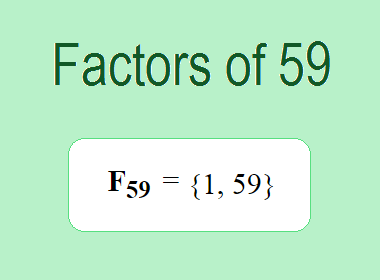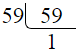# Factors of 59The factors of 59 are 1 and 59 i.e. F59 = {1, 59}. The factors of 59 are all the numbers that can divide 59 without leaving a remainder. 59 is a prime number, so it is divisible by 1 and 59 only.

We can check if these numbers are factors of 59 by dividing 59 by each of them. If the result is a whole number, then the number is a factor of 59. Let's do this for each of the numbers listed above:

·        1 is a factor of 59 because 59 divided by 1 is 59.

·        59 is a factor of 59 because 59 divided by 59 is 1.

## Properties of the Factors of 59

The factors of 59 have some interesting properties. One of the properties is that the sum of the factors of 59 is equal to 60. We can see this by adding all the factors of 59 together:

1 + 59 = 60

Another property of the factors of 59 is that the only prime factor of 59 is 59 itself.

********************

********************

## Applications of the Factors of 59

The factors of 59 have several applications in mathematics. One of the applications is in finding the highest common factor (HCF) of two or more numbers. The HCF is the largest factor that two or more numbers have in common. For example, to find the HCF of 59 and 118, we need to find the factors of both numbers and identify the largest factor they have in common. The factors of 59 are 1, and 59. The factors of 118 are 1, 2, 59, and 118. The largest factor that they have in common is 59. Therefore, the HCF of 59 and 118 is 59.

Another application of the factors of 59 is in prime factorization. Prime factorization is the process of expressing a number as the product of its prime factors. The prime factor of 59 is 59 since it is only the prime number that can divide 59 without leaving a remainder. Therefore, we can express 59 as:

59 = 59

We can do prime factorization by division method as given below,59 = 59

Since 59 is a prime number, there is no factor tree of 59.

## Conclusion

The factors of 59 are the numbers that can divide 59 without leaving a remainder. The factors of 59 are 1 and 59. The factors of 59 have some interesting properties, such as having a sum of 60. The factors of 59 have several applications in mathematics, such as finding the highest common factor and prime factorization.